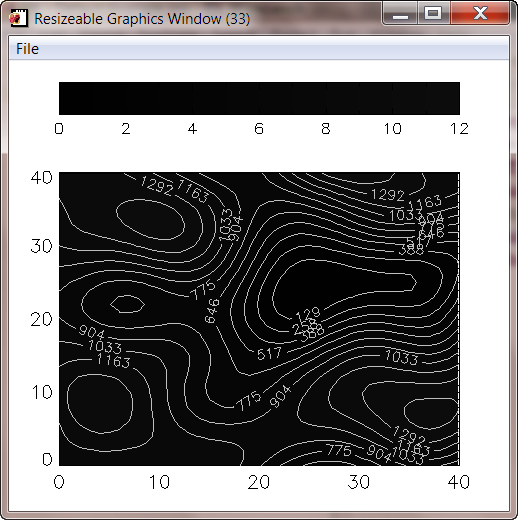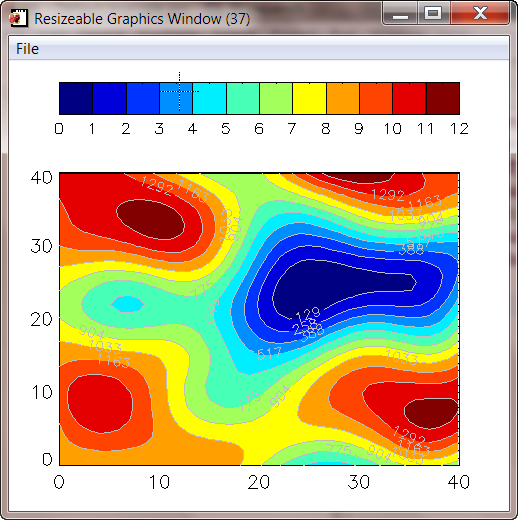# Using Colors in cgWindow

QUESTION: I have a program using a cgWindow resizeable graphics window, but I have to run it twice to get the colors correct. What am I doing wrong?

Here is the program I am running.

```   PRO Example
cgWindow, wxsize=500, wysize=450
cgContour, cgDemoData(2), NLevels=12, Position=[0.1, 0.1, 0.9, 0.75], \$
cgContour, cgDemoData(2), NLevels=12, /Overplot, Color='gray', /Add
END
```

And here is the result the first time I run the program. When I run it again, the colors are perfect. What is happening here?The colors are incorrect the first time the program is run.ANSWER: The problem here is the way you are loading colors into cgWindow. The cgWindow program actually protects its colors so that the graphic output is always displayed with the proper colors, no matter what colors are currently loaded in the hardware color table. The cgWindow program obtains its color vectors from the current hardware color table when the program is first called. In your case, you haven't loaded any colors into the color table when you first call cgWindow, so the colors that are loaded into the window are the normal grayscale color table values.

Your second command loads colors into the hardware color table, but too late to affect the way cgWindow will use the colors. However, the next time you run the program, the proper colors are already loaded when cgWindow starts up, so it uses the colors you expect.

You can solve this problem in either of two ways. First, you can simply load the colors you want to use in cgWindow before you call cgWindow, like this.

```   PRO Example
cgWindow, wxsize=500, wysize=450
cgContour, cgDemoData(2), NLevels=12, Position=[0.1, 0.1, 0.9, 0.75], \$
cgContour, cgDemoData(2), NLevels=12, /Overplot, Color='gray', /Add
END
```

Or, you can “send” the colors you are loading to cgWindow by setting the Window keyword on the cgLoadCT command, like this.

```   PRO Example
cgWindow, wxsize=500, wysize=450
cgContour, cgDemoData(2), NLevels=12, Position=[0.1, 0.1, 0.9, 0.75], \$
cgContour, cgDemoData(2), NLevels=12, /Overplot, Color='gray', /Add
END
```

Either method will produce a display with the correct colors.The colors are correct if the colors are loaded properly.